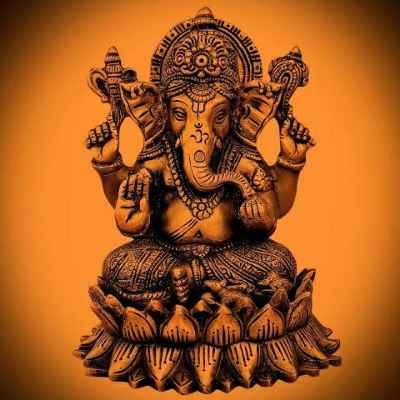Forums

# Receivables

In illustration 18 , (mosaic ltd question) the total cost of the proposed policy is 75% of sales= 1293750.variable cost is 80% of 75% of sales = 1035000 & fixed cost = 225000 (since it did not change ) But total cost is variable + fixed cost rite But when we add variable + fixed , it is not equal to 1293750, then what the balance indicate ? Is there even a balance ? Can u please reply?Swathi Krishna

CA Inter

7K+

26-Jul-20

50

This is from working capital sectionSwathi Krishna

CA Inter

7K+

26-Jul-20

Let us take an example to understand your query. Suppose the fixed cost is Rs.2,00,000 at 1,00,000 units and variable cost is say Rs.10 per unit i.e., variable cost = Rs.10 x 1,00,000 units = Rs.10,00,000. Therefore, total cost = Fixed cost + Variable cost = Rs.2,00,000 + Rs.10,00,000 = Rs.12,00,000 When we increase the production from 1,00,000 units to 1,50,000 units, fixed cost being fixed in nature like the rent which do not change with production remains the same i.e., it will be Rs.2,00,000 at 1,50,000 units also. But coming to variable cost it is fixed at Rs.10 per unit but varies in total because it is calculated on the output basis. At 1,50,000 units, Variable cost = 1,50,000 units x Rs.10 = Rs.15,00,000. Total cost at 1,50,000 units will be Fixed cost + Variable cost = Rs.2,00,000 + Rs.15,00,000 = Rs.17,00,000. So at 1,00,000 units the total cost is Rs.12,00,000 and at 1,50,000 units it is Rs.17,00,000. There is no point of balance in total cost. Just remember one thing that Fixed cost is always fixed in total and varies per unit whereas Variable cost is fixed per unit and varies in total.CA Inter

2K+

27-Jul-20

So after increase the total cost would 1260000Swathi Krishna

CA Inter

7K+

27-Jul-20

That is the percentage of the variable in the total cost would remain same since the per unit of variable cost is constant in the total cost per unit. Percentage of fixed cost in total cost is varying rite that is per unit of fixed cost is changing Is this what u mean?Swathi Krishna

CA Inter

7K+

27-Jul-20

Swathi Krishna

That is the percentage of the variable in the total cost would remain same since the per unit of variable cost is constant in the total cost per unit. Percentage of fixed cost in total cost is varying rite that is per unit of fixed cost is changing Is this what u mean?

Total cost = Fixed Cost + Variable cost. So if % of Fixed cost in total cost comes down it means % of variable cost in total cost goes up Eg: 100% = 40% +60% if one goes up other comes downSriram Somayajula

28-Jul-20

In above question the total cost =1725000*75% = 1293750 ,then vc=1293750*80%= 1035000 and balance should be fixed cost rite But why is fixed cost= 225000 ?Swathi Krishna

CA Inter

7K+

28-Jul-20

At Rs.15,00,000 sales, Cost of sales is given to be 75%. Out of which 80% is towards variable costs and 20% is towards Fixed costs. So at 15,00,000 sale point, variable cost will be 9,00,000 (15,00,000 x 75% x 80%). And Fixed cost will be 2,25,000 (15,00,000 x 75% x 20%). When the sales will be increased by 15%, amount of sales = 17,25,000 (15,00,000 + 15%). At this point variable cost will be 10,35,000 (9,00,000 + 15%). But the fixed cost being fixed in nature do not change even when the sales increase by 15% and will remain at Rs.2,25,000.CA Inter

2K+

31-Jul-20

Sales